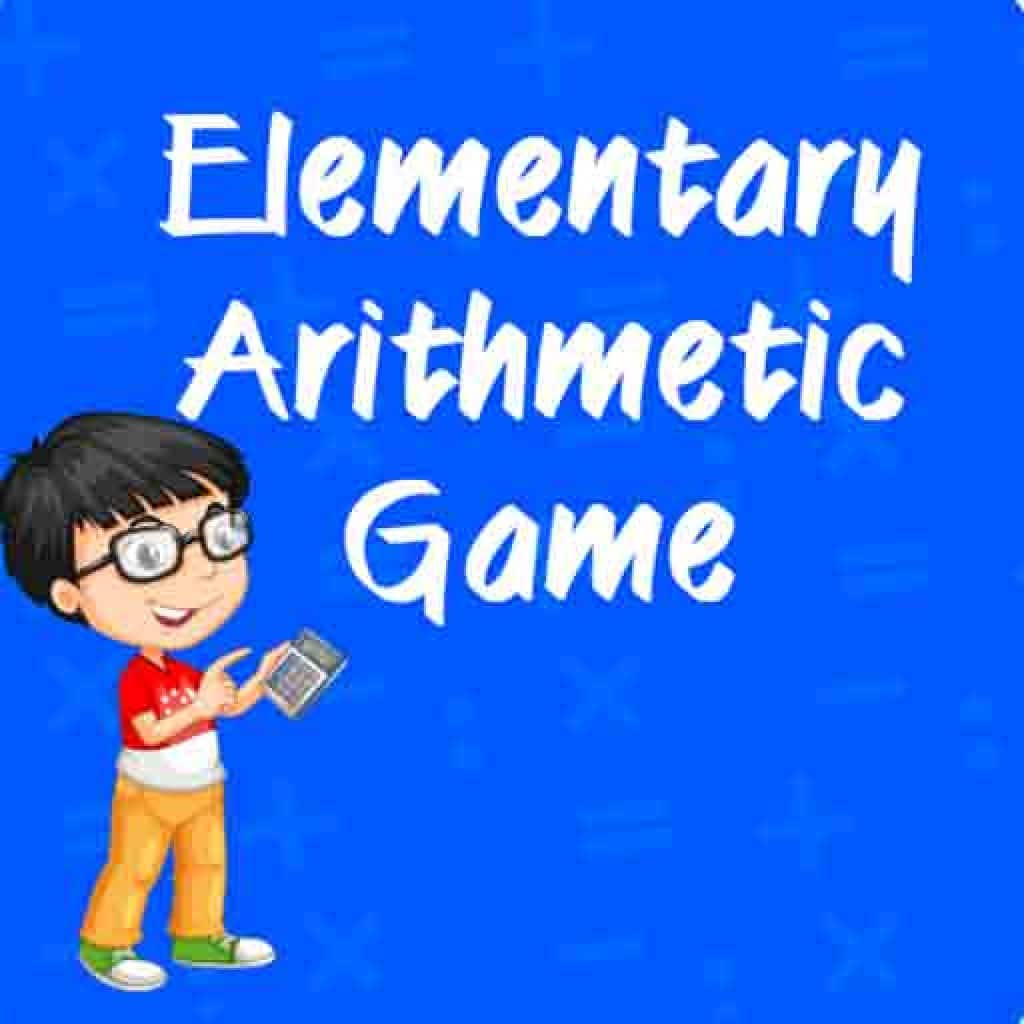# Elementary Arithmetic Game

You have 60 seconds to complete a many equation as possible. In each equation, the elementary arithmetic is missing and you have to choose the right one based on the numbers and answer and complete the equation.